# How to get values of an NumPy array at certain index positions?

• Last Updated : 25 Oct, 2020

Sometimes we need to remove values from the source Numpy array and add them at specific indices in the target array. In NumPy, we have this flexibility, we can remove values from one array and add them to another array. We can perform this operation using numpy.put() function and it can be applied to all forms of arrays like 1-D, 2-D, etc.

Example 1:

## Python3

 `# Importing Numpy module``import` `numpy as np`` ` `# Creating 1-D Numpy array``a1 ``=` `np.array([``11``, ``10``, ``22``, ``30``, ``33``])``print``(``"Array 1 :"``)``print``(a1)`` ` `a2 ``=` `np.array([``1``, ``15``, ``60``])``print``(``"Array 2 :"``)``print``(a2)`` ` `print``("\nTake ``1` `and` `15` `from` `Array ``2` `and` `put them ``in``\``1st` `and` `5th` `position of Array ``1``")`` ` `a1.put([``0``, ``4``], a2)`` ` `print``(``"Resultant Array :"``)``print``(a1)`

Output: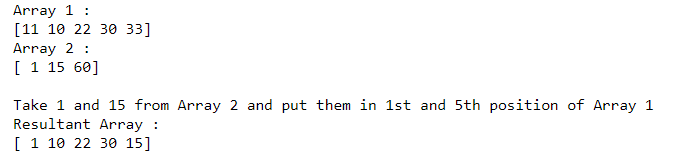In the above example, we take two 1-D arrays and transfer values from one array to another at specific positions.

Example 2:

## Python3

 `# Importing Numpy module``import` `numpy as np`` ` `# Creating 2-D Numpy array``a1 ``=` `np.array([[``11``, ``10``, ``22``, ``30``],``               ``[``14``, ``58``, ``88``, ``100``]])`` ` `print``(``"Array 1 :"``)``print``(a1)`` ` `a2 ``=` `np.array([``1``, ``15``, ``6``, ``40``])``print``(``"Array 2 :"``)``print``(a2)`` ` `print``("\nTake ``1``, ``15` `and` `6` `from` `Array ``2` `and` `put them ``in` `1st``,\``4th` `and` `7th` `positions of Array ``1``")`` ` `a1.put([``0``, ``3``, ``6``], a2)`` ` `print``(``"Resultant Array :"``)``print``(a1)`

Output: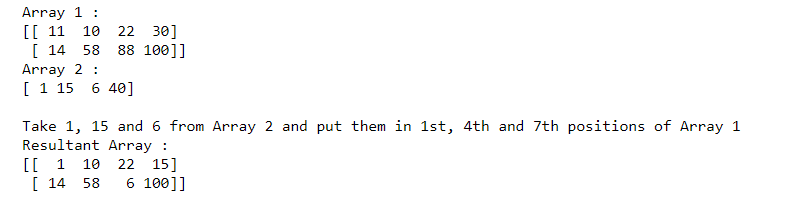In the above example, we take two different arrays and transfer values from 1-D to 2-D array at specific positions.

Example 3:

## Python3

 `# Importing Numpy module``import` `numpy as np`` ` `# Creating 3-D Numpy array``a1 ``=` `np.array([[[``11``, ``25``, ``7``], [``30``, ``45``, ``55``], [``20``, ``45``, ``7``]],``               ``[[``50``, ``65``, ``8``], [``70``, ``85``, ``10``], [``11``, ``22``, ``33``]],``               ``[[``19``, ``69``, ``36``], [``1``, ``5``, ``24``], [``4``, ``20``, ``9``]]])`` ` ` ` `print``(``"Array 1 :"``)``print``(a1)`` ` `# Creating 2-D array``a2 ``=` `np.array([[``1``, ``15``, ``10``],``               ``[``6``, ``40``, ``50``],``               ``[``11``, ``5``, ``10``]])`` ` `print``(``"\nArray 2 :"``)``print``(a2)`` ` `print``("\nTake ``1``, ``15``, ``10``, ``6``, ``40` `and` `50` `from` `Array ``2` `and` `put\``them ``in` `1st``, ``3rd``, ``5th``, ``9th``, ``11th` `and` `15th` `positions of Array ``1``")`` ` `a1.put([``0``, ``2``, ``4``, ``8``, ``10``, ``14``], a2)`` ` `print``(``"Resultant Array :"``)``print``(a1)`

Output: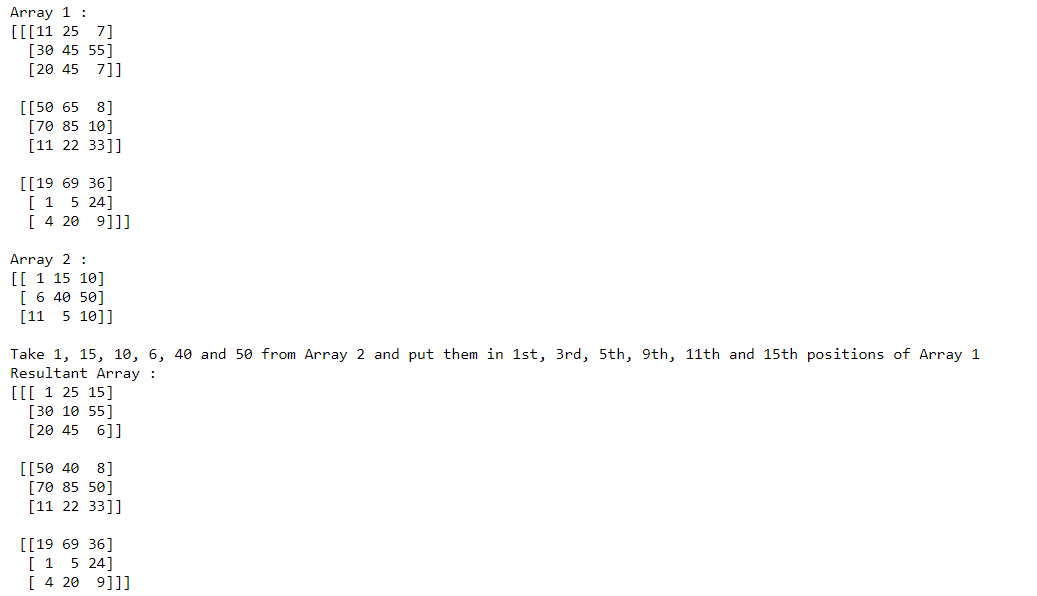In the above example, we take two different arrays and transfer values from 2-D to 3-D array at specific positions.   One question arises, what will happen if out-of-bounds indices occur. For this, we have 3 modes in numpy.put() function

mode = {‘raise’, ‘wrap’, ‘clip’}

• ‘raise’ – raise an error (default)
• ‘wrap’ – wrap around
• ‘clip’ – clip to the range

Example 4: Taking mode = ‘raise’

## Python3

 `# Importing Numpy module``import` `numpy as np`` ` `# Creating 2-D Numpy array``a1 ``=` `np.array([[``11``, ``10``, ``22``],``               ``[``14``, ``58``, ``88``]])`` ` `print``(``"Array 1 :"``)``print``(a1)`` ` `a2 ``=` `np.array([[``1``, ``15``, ``6``],``               ``[``40``, ``50``, ``70``]])`` ` `print``(``"Array 2 :"``)``print``(a2)`` ` `print``("\nTake ``1` `and` `15` `from` `Array ``2` `and` `put them ``in` `1st` `\``and` `5th` `positions of Array ``1``")`` ` `print``("by mistake we write the index which ``is` `out of bound,\``now mode will play its role")`` ` `a1.put([``0``, ``15``], a2, mode``=``'raise'``)`` ` `print``(``"\nResultant Array :"``)``print``(a1)`

Output: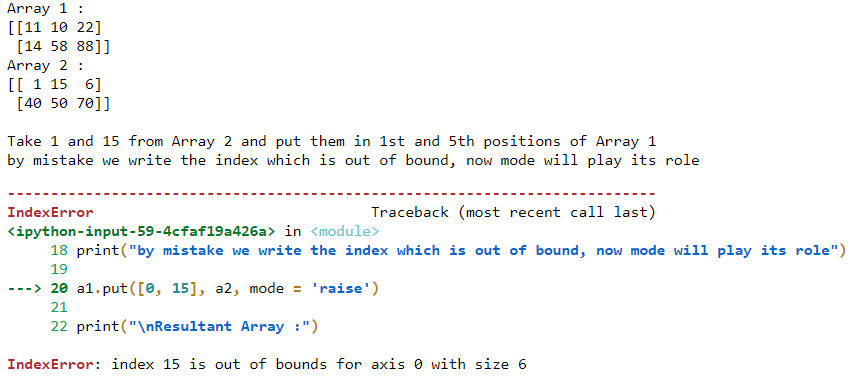In the above example mode=’raise’ generates an error when indices become out of bound.

Example 5: Taking mode = ‘clip’

## Python3

 `# Importing Numpy module``import` `numpy as np`` ` `# Creating 2-D Numpy array``a1 ``=` `np.array([[``11``, ``10``, ``22``],``               ``[``14``, ``58``, ``88``]])`` ` `print``(``"Array 1 :"``)``print``(a1)`` ` `a2 ``=` `np.array([[``1``, ``15``, ``6``],``               ``[``40``, ``50``, ``70``]])`` ` `print``(``"Array 2 :"``)``print``(a2)`` ` `print``("\nTake ``1` `and` `15` `from` `Array ``2` `and` `put them ``in` `1st` `and``\``5th` `positions of Array ``1``")`` ` `print``("by mistake we write the index which ``is` `out of bound,\``now mode will play its role")`` ` `a1.put([``0``, ``15``], a2, mode ``=` `'clip'``)`` ` `print``(``"\nResultant Array :"``)``print``(a1)`

Output: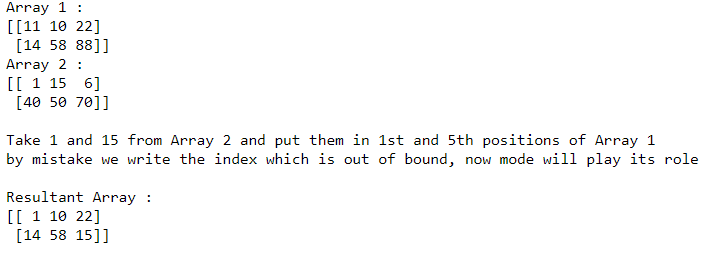In the above example, mode=’clip’ replaces the last element along the axis instead of raising any error.

My Personal Notes arrow_drop_up